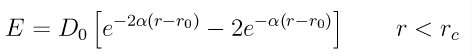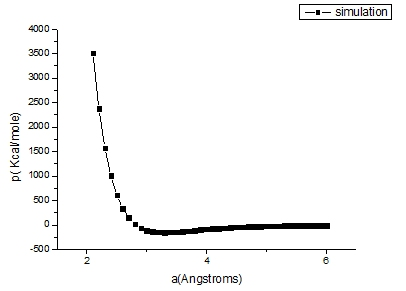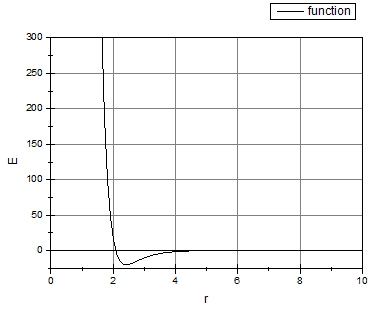# test potential

Dear Lammps users,
I write my own pair potentials and I want to check if the equation is corrected coded in the potential files, so at first I need to find a good checking method.
Take morse potential for example,,and I set D0=19.7866, alpha=2.0579 and r0=2.4200 for Mg, then I minimize the energy of the system for different initial lattice constant at 0K, and output lattice constant a and per-atom potential energy P, the comparison is like this:I find out that they differ much. Is my method reasonable? or how can I do the simulation to get the second line so as to check my own potential code?

Any help will be appreciated, thank you so much.
Best regards,
Michelle

Dear Lammps users,
I write my own pair potentials and I want to check if the equation is
corrected coded in the potential files, so at first I need to find a good
checking method.
Take morse potential for example, ,and I set D0=19.7866, alpha=2.0579 and
r0=2.4200 for Mg, then I minimize the energy of the system for different
initial lattice constant at 0K, and output lattice constant a and per-atom
potential energy P, the comparison is like this:
I find out that they differ much. Is my method reasonable? or how can I
do the simulation to get the second line so as to check my own potential
code?

​the simplest test is to use the "pair_write" command and then plot the
potential and force as written out and compare to your formula. however,
that tests only the Pair::single() API and not Pair::compute()​

for the latter, you could do a series of calculations with two atoms that
you place at different distances with a loop and then compute the energy
and force via "run 0".

this kind of topic has come up recently in the mailing list. please check
out https://sourceforge.net/p/lammps/mailman/message/34925329/ . the
specific testing example comes up somewhere in the middle of the thread.

axel.